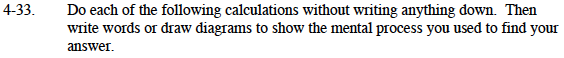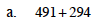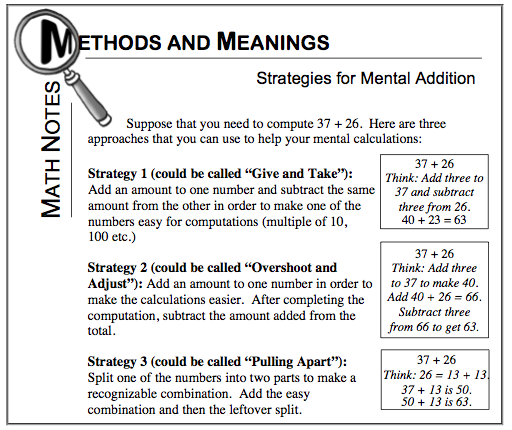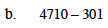### Home > MC1 > Chapter 4 > Lesson 4.1.3 > Problem4-33

4-33.

Do each of the following calculations without writing anything down. Then write words or draw diagrams to show the mental process you used to find your answer.

1. 491 + 294

2. 4710 − 301Look at the Math Notes box from Lesson 3.1.2 on the below.
Is there one strategy for mental addition that works best for you?Olga used the ''Overshoot and Adjust'' strategy for this problem.
She thought, add 6 to 294 to make 300, then 491 + 300 = 791.

That does make this addition easier, but don't forget the last step!
Olga still needs to subtract 6 in order to ''adjust'' her answer.

How did you solve this problem? Make sure to explain your own mental process!Addition and subtraction are very similar!
Use the Math Notes box again to solve this problem, but remember to use subtraction.

The difference is 4409. Did you show your mental process?